The partial derivative functions ddx, ddy and fwidth are some of the least used hlsl functions and they look quite confusing at first, but I like them a lot and I think they have some straightforward useful use cases so I hope I can explain them to you. Since I’m explaining straightforward functions you don’t have to know a lot of shader programming for this, but you should have a rough overview over how to render simple things with shaders in unity. If don’t know the basics yet, I have a couple of tutorials on them here.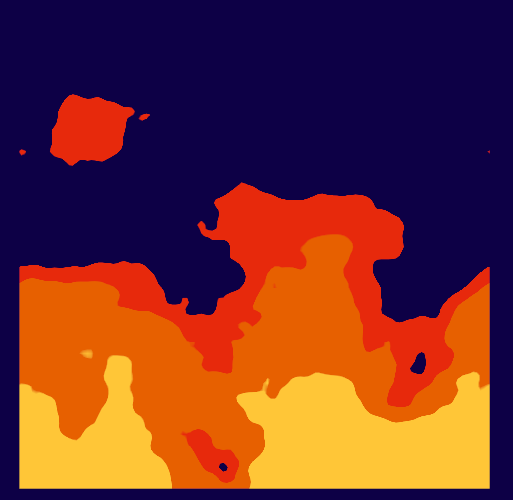## DDX and DDY

“Derivative” is a fancy word which means “change of a function” at a point. In this case we can use any value and get the change between the neighboring speenspace pixels. `ddx` and `ddy` are the simpler 2 of the 3 functions, they compare values of two pixels next to each other vertically or horizontally. This isn’t something that’s possible with any other functions and relies on a special architetecture detail of the GPU you might not expect. Instead of calculating every pixel completely on their own, pixels are grouped in little 2x2 fields that are calculated in parallel and in those units any information can be compared. The ddx function returns the value of the subtraction of the left pixel of a horizontal pixel pair from the right pixel. The ddy pixel works similarly for the vertical axis. This means that two pixels in such a pixel pair always return the same value for ddx or ddy.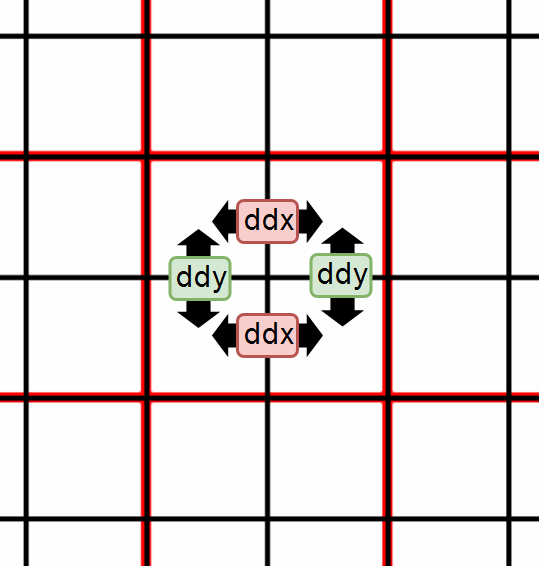For a test I used a simple shader with UV coordinates and returned the derivative of the first component of the coordinate multiplied by an adjustable factor. The `.xxx` I used converts the 1d scalar value to a 3d value with the same value for all 3 components.

``````//the fragment shader
fixed4 frag(v2f i) : SV_TARGET{
//calculate the change of the uv coordinate to the next pixel
float derivative = ddx(i.uv.x) * _Factor;
//transform derivative to greyscale color
fixed4 col = float4(derivative.xxx , 1);
col *= _Color;
return col;
}
``````

When we now play around with this shader we can see that it changes color depending on how much the x value of the UVs changes in relation to the screen x pixels. If we zoom closer to the surface or scale it up theres less change per pixel and the surface gets darker. If we rotate the surface the change in uv.x is in the screen y axis instead of the x axis and the surface becomes again darker.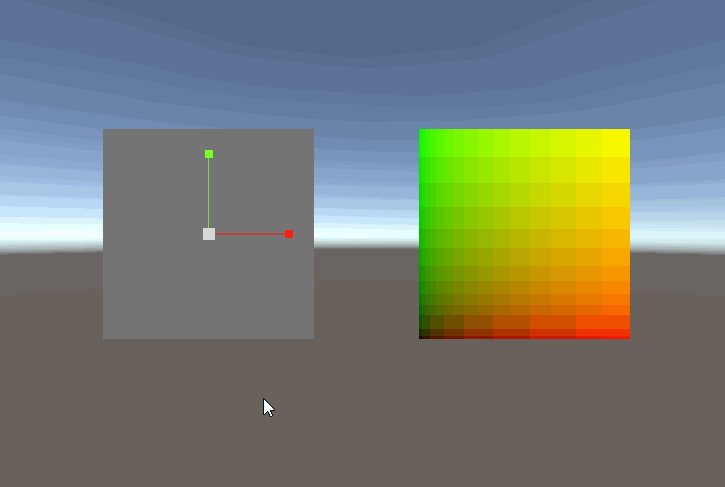This alone can be very powerful. For example it’s possible to very quickly calculate low-quality normalmaps from depth maps from this and the tex2D function uses this internally to choose between mipmap levels. But the most frequent use I have needs the overall change of a value, not the directional one, this is what fwidth gives us.

## fwidth

If we want to combine ddx and ddy the most straightforward way to do that is to get their absolute values and then add them, so a custom implementation would look like this (you don’t have to add this code to your shader since the internal definition of fwidth already does this):

``````float fwidth(float value){
return abs(ddx(value)) + abs(ddy(value));
}
``````

If we replace the ddx in our test shader from earlier with an fwidth we can see that zooming or scaling still has the same effect, but rotating now only changes the brightness slightly, having the same grey at 90° angles, but being a bit brighter inbetween. We could eliminate the color change by writing our own fwidth function with a little bit of fancy trigonometry (we’d square the results of ddx and ddy and take the square root of the sum), but in most cases the higher quality of the math here isn’t worth the performance hit of the more complicated math.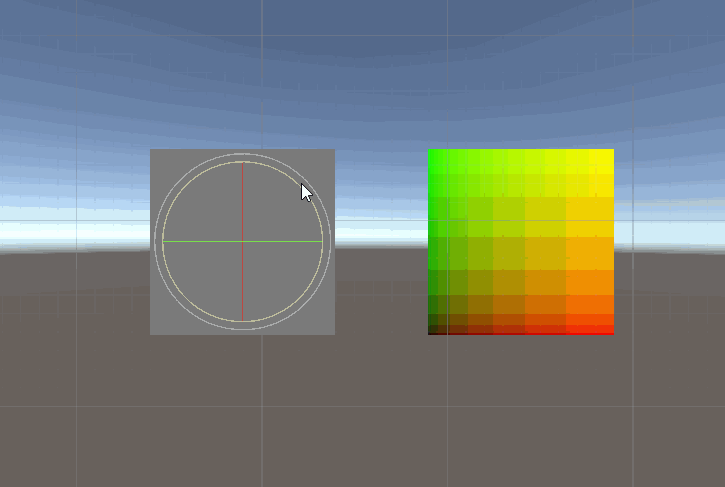## Non-aliased step

The #1 usecase for fwidth (at least for me) is to cut off gradients at a specific value into distinct fields without getting aliasing artefacts, this is used in many variations for effects like fire, water, toon lighting and many more. The most straightforward way to cut off a gradient this way is to take the step of the gradient value and the cutoff value and then do a linear interpolation with that step result from the color of one side to the color of the other side. This step introduces aliasing though, jaggy edges we usually want to avoid. The way to avoid this is to do a inverse lerp based on how much the value changes over a single pixel.

We start doing the non aliased step by first calculating the fwidth value of our gradient (I’ll use the UV x component here again, but anything works, try around what you can get away with!). Since the next step is to do the inverse lerp from half a pixel before the cutoff value to half a pixel after the cutoff value to get a whole pixel gradient we also divide the change by 2 here.

After successfully calculating half of the change, we can do the inverse lerp. Instead of that I also often use the smoothstep function since it’s a built-in function, but that one also does some smoothing we don’t need here, so it’s less effective overall. The inverse of a interpolation means that the calculation returns 0 if the input is equal to the first value or 1 if it’s equal to the second value and it returns the inbetween values as expected. To get it we subtract the lower edge of the range from the value, this moves the 0 intersection to the correct value. Then we divide by the difference between the lower to the upper edge of the function. Because this process allows input values outside of the specified edges we end the calculation by clamping the result between 0 and 1 with the saturate function.

``````//the fragment shader
fixed4 frag(v2f i) : SV_TARGET{
//you can use almost any value as a gradient
//calculate the change
float halfChange = fwidth(gradient) / 2;
//base the range of the inverse lerp on the change over one pixel
float lowerEdge = 0.5 - halfChange;
float upperEdge = 0.5 + halfChange;
//do the inverse interpolation
float stepped = (gradient - lowerEdge) / (upperEdge - lowerEdge);
stepped = saturate(stepped);
//convert to greyscale color for output
fixed4 col = float4(stepped.xxx, 1);
return col;
}
``````

Here I compare the regular step function to the non aliased step we just wrote as well as the one that uses the `smoothstep` function. On the left surface you can see the aliasing jaggyness of the step function while the other two functions provide a smoother transition. I also can’t make out a definitive difference between the smoothstep version and the cheaper inverse lerp so I recommend you to stick with that instead of the builtin function.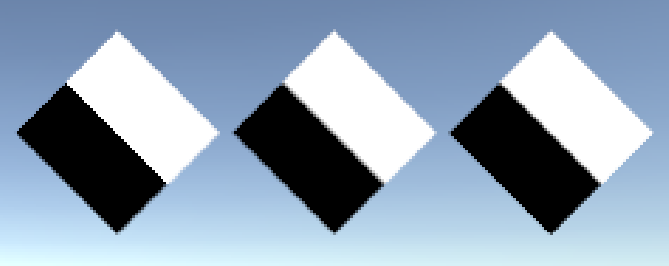## A better step?

So far we can see better results with the new technique, but it’s also kind of bothersome to write and a bit slower. We can’t change the performance demands of the functions but I’d also argue that in 99.9% of all cases your performance bottleneck won’t be here, as mentioned previously every tex2d call also accesses those functions and thats by far not expensive part of a texture sample. What we can do is to write a custom function that’s easy to use as `step` and can work as a drag and drop replacement.

Step returns 1 if the first argument is smaller than the second and 0 otherwise. We’ll translate those arguments into the comparison value as the first argument and the gradient value as the second one and then we translate the code of the previous implementation into a function that only depends on those two arguments.

``````//smooth version of step
float halfChange = fwidth(gradient) / 2;
//base the range of the inverse lerp on the change over one pixel
float lowerEdge = compValue - halfChange;
float upperEdge = compValue + halfChange;
//do the inverse interpolation
float stepped = (gradient - lowerEdge) / (upperEdge - lowerEdge);
stepped = saturate(stepped);
return stepped;
}

fixed4 frag(v2f i) : SV_TARGET{
float stepped = aaStep(0.5, i.uv.x);
//value to greyscale color with full alpha
fixed4 col = float4(stepped.xxx, 1);
return col;
}
``````

## An example

One nice use for step is to make procedural fire. I based this example loosely on Febucci’s fire shader.

We shift the texture UVs based on the time, and read from a noise texture, as the gradient how “intense” a fire is at any position, I’ll use a square of the inverse uv y component as that gets us a good amount fire with my noise texture (I used layered perlin noise, generated via the texture baking tool I made a tutorial about). Then I generated the cutoff values for the texture, for the shape I used the step between the noise texture and the gradient and for the edges between the colors I did the same but with some offset based on adjustable properties. To combine those colors we can start by making everything the “outer” color and then interpolating to the “inner” colors wherever they are visible.

I also modified the aaStep here to interpolate over 2 pixels instead of one by not dividing the result of the fwidth function by 2, this is something you can play around with and see what feels best for your use case.

``````//smooth version of step
//base the range of the inverse lerp on the change over two pixels
float lowerEdge = compValue - change;
float upperEdge = compValue + change;
//do the inverse interpolation
float stepped = (gradient - lowerEdge) / (upperEdge - lowerEdge);
stepped = saturate(stepped);
//smoothstep version here would be `smoothstep(lowerEdge, upperEdge, gradient)`
return stepped;
}

fixed4 frag(v2f i) : SV_TARGET{
//I square this here to make the fire look a bit more "full"
float fireGradient = 1 - i.uv.y;
//calculate fire UVs and animate them
float2 fireUV = TRANSFORM_TEX(i.uv, _MainTex);
fireUV.y -= _Time.y * _ScrollSpeed;
//get the noise texture
float fireNoise = tex2D(_MainTex, fireUV).x;

//calculate whether fire is visibe at all and which colors should be shown
float edge1 = aaStep(fireNoise, fireGradient - _Edge1);
float edge2 = aaStep(fireNoise, fireGradient - _Edge2);

//define shape of fire
fixed4 col = _Color1 * outline;
col = lerp(col, _Color2, edge1);
col = lerp(col, _Color3, edge2);

//uv to color
return col;
}
``````Here is a comparison of step vs. the new non aliased step. It’s not huge and if you have a pixely aesthetic it makes your game look worse, but I think it’s a good step to making your game look a little better, especially when you have a soft aesthetic and want the game to also look smooth at low-ish resolutions (along the lines of 720p, not pixel art).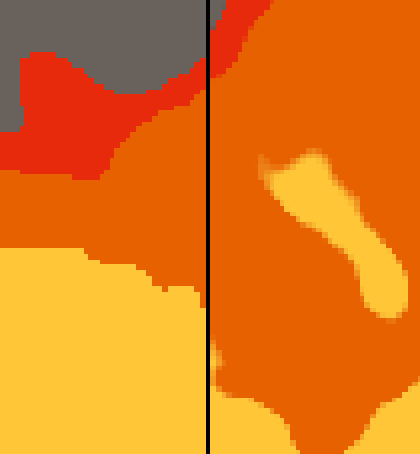## Sources

``````Shader "Tutorial/046_Partial_Derivatives/testing"{
//show values to edit in inspector
Properties{
_Factor("Factor", Range(0, 100)) = 1
}

//the material is completely non-transparent and is rendered at the same time as the other opaque geometry
Tags{ "RenderType"="Opaque" "Queue"="Geometry"}

Cull Off

Pass{
CGPROGRAM

#include "UnityCG.cginc"

#pragma vertex vert
#pragma fragment frag

float _Factor;

//the object data that's put into the vertex shader
struct appdata{
float4 vertex : POSITION;
float2 uv : TEXCOORD0;
};

//the data that's used to generate fragments and can be read by the fragment shader
struct v2f{
float4 position : SV_POSITION;
float2 uv : TEXCOORD0;
};

v2f vert(appdata v){
v2f o;
//convert the vertex positions from object space to clip space so they can be rendered
o.position = UnityObjectToClipPos(v.vertex);
o.uv = v.uv;
return o;
}

fixed4 frag(v2f i) : SV_TARGET{
//calculate the change of the uv coordinate to the next pixel
float derivative = fwidth(i.uv.x) * _Factor;
//transform derivative to greyscale color
fixed4 col = float4(derivative.xxx , 1);
return col;
}

ENDCG
}
}
}
``````
``````Shader "Tutorial/046_Partial_Derivatives/aaStep"{
//show values to edit in inspector
Properties{

}

//the material is completely non-transparent and is rendered at the same time as the other opaque geometry
Tags{ "RenderType"="Opaque" "Queue"="Geometry"}

Cull Off

Pass{
CGPROGRAM

#include "UnityCG.cginc"

#pragma vertex vert
#pragma fragment frag

//the object data that's put into the vertex shader
struct appdata{
float4 vertex : POSITION;
float2 uv : TEXCOORD0;
};

//the data that's used to generate fragments and can be read by the fragment shader
struct v2f{
float4 position : SV_POSITION;
float2 uv : TEXCOORD0;
};

v2f vert(appdata v){
v2f o;
//convert the vertex positions from object space to clip space so they can be rendered
o.position = UnityObjectToClipPos(v.vertex);
o.uv = v.uv;
return o;
}

//smooth version of step
float halfChange = fwidth(gradient) / 2;
//base the range of the inverse lerp on the change over one pixel
float lowerEdge = compValue - halfChange;
float upperEdge = compValue + halfChange;
//do the inverse interpolation
float stepped = (gradient - lowerEdge) / (upperEdge - lowerEdge);
stepped = saturate(stepped);
//smoothstep version here would be `smoothstep(lowerEdge, upperEdge, gradient)`
return stepped;
}

fixed4 frag(v2f i) : SV_TARGET{
float stepped = aaStep(0.5, i.uv.x);
//value to greyscale color with full alpha
fixed4 col = float4(stepped.xxx, 1);
return col;
}

ENDCG
}
}
}
``````
``````Shader "Tutorial/046_Partial_Derivatives/fire"{
//show values to edit in inspector
Properties{
_MainTex ("Fire Noise", 2D) = "white" {}
_ScrollSpeed("Animation Speed", Range(0, 2)) = 1

_Color1 ("Color 1", Color) = (0, 0, 0, 1)
_Color2 ("Color 2", Color) = (0, 0, 0, 1)
_Color3 ("Color 3", Color) = (0, 0, 0, 1)

_Edge1 ("Edge 1-2", Range(0, 1)) = 0.25
_Edge2 ("Edge 2-3", Range(0, 1)) = 0.5
}

//the material is completely non-transparent and is rendered at the same time as the other opaque geometry
Tags{ "RenderType"="transparent" "Queue"="transparent"}

Cull Off
Blend SrcAlpha OneMinusSrcAlpha
ZWrite Off

Pass{
CGPROGRAM

#include "UnityCG.cginc"

#pragma vertex vert
#pragma fragment frag

//tint of the texture
fixed4 _Color1;
fixed4 _Color2;
fixed4 _Color3;

float _Edge1;
float _Edge2;

float _ScrollSpeed;

sampler2D _MainTex;
float4 _MainTex_ST;

//the object data that's put into the vertex shader
struct appdata{
float4 vertex : POSITION;
float2 uv : TEXCOORD0;
};

//the data that's used to generate fragments and can be read by the fragment shader
struct v2f{
float4 position : SV_POSITION;
float2 uv : TEXCOORD0;
};

v2f vert(appdata v){
v2f o;
//convert the vertex positions from object space to clip space so they can be rendered
o.position = UnityObjectToClipPos(v.vertex);
o.uv = v.uv;
return o;
}

//smooth version of step
//base the range of the inverse lerp on the change over two pixels
float lowerEdge = compValue - change;
float upperEdge = compValue + change;
//do the inverse interpolation
float stepped = (gradient - lowerEdge) / (upperEdge - lowerEdge);
stepped = saturate(stepped);
//smoothstep version here would be `smoothstep(lowerEdge, upperEdge, gradient)`
return stepped;
}

fixed4 frag(v2f i) : SV_TARGET{
//I square this here to make the fire look a bit more "full"
float fireGradient = 1 - i.uv.y;
//calculate fire UVs and animate them
float2 fireUV = TRANSFORM_TEX(i.uv, _MainTex);
fireUV.y -= _Time.y * _ScrollSpeed;
//get the noise texture
float fireNoise = tex2D(_MainTex, fireUV).x;

//calculate whether fire is visibe at all and which colors should be shown
float edge1 = aaStep(fireNoise, fireGradient - _Edge1);
float edge2 = aaStep(fireNoise, fireGradient - _Edge2);

//define shape of fire
fixed4 col = _Color1 * outline;
col = lerp(col, _Color2, edge1);
col = lerp(col, _Color3, edge2);

//uv to color
return col;
}

ENDCG
}
}
}
``````

You can also find me on twitter at @totallyRonja. If you liked my tutorial and want to support me you can do that on Patreon (patreon.com/RonjaTutorials) or Ko-Fi (ko-fi.com/RonjaTutorials).